# How to convert time into decimals in Excel

You can watch a video tutorial here.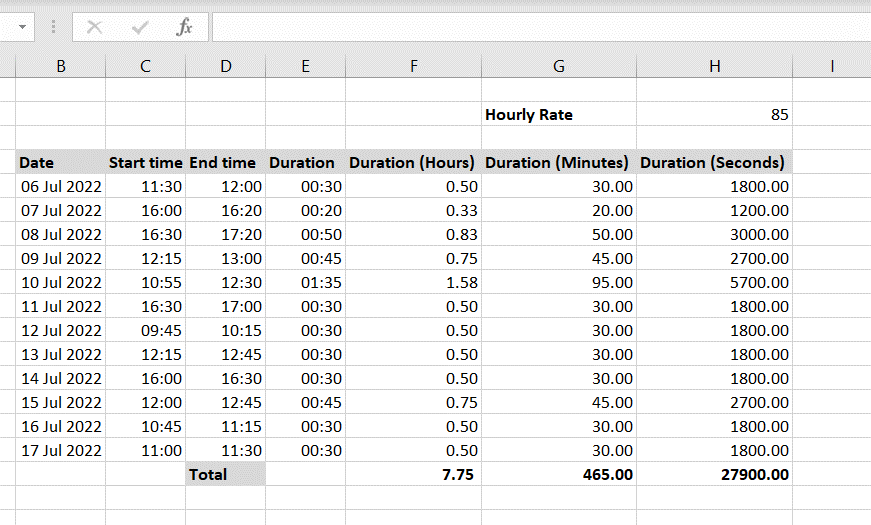To perform calculations with time or duration displayed in the time format, it needs to be converted into decimals or into the number format. For example, if you need to compute the cost of a task in which the duration is recorded in hours, the number will have to be converted into a number to be multiplied by the hourly rate.

### Step 1 – Multiply the value by 24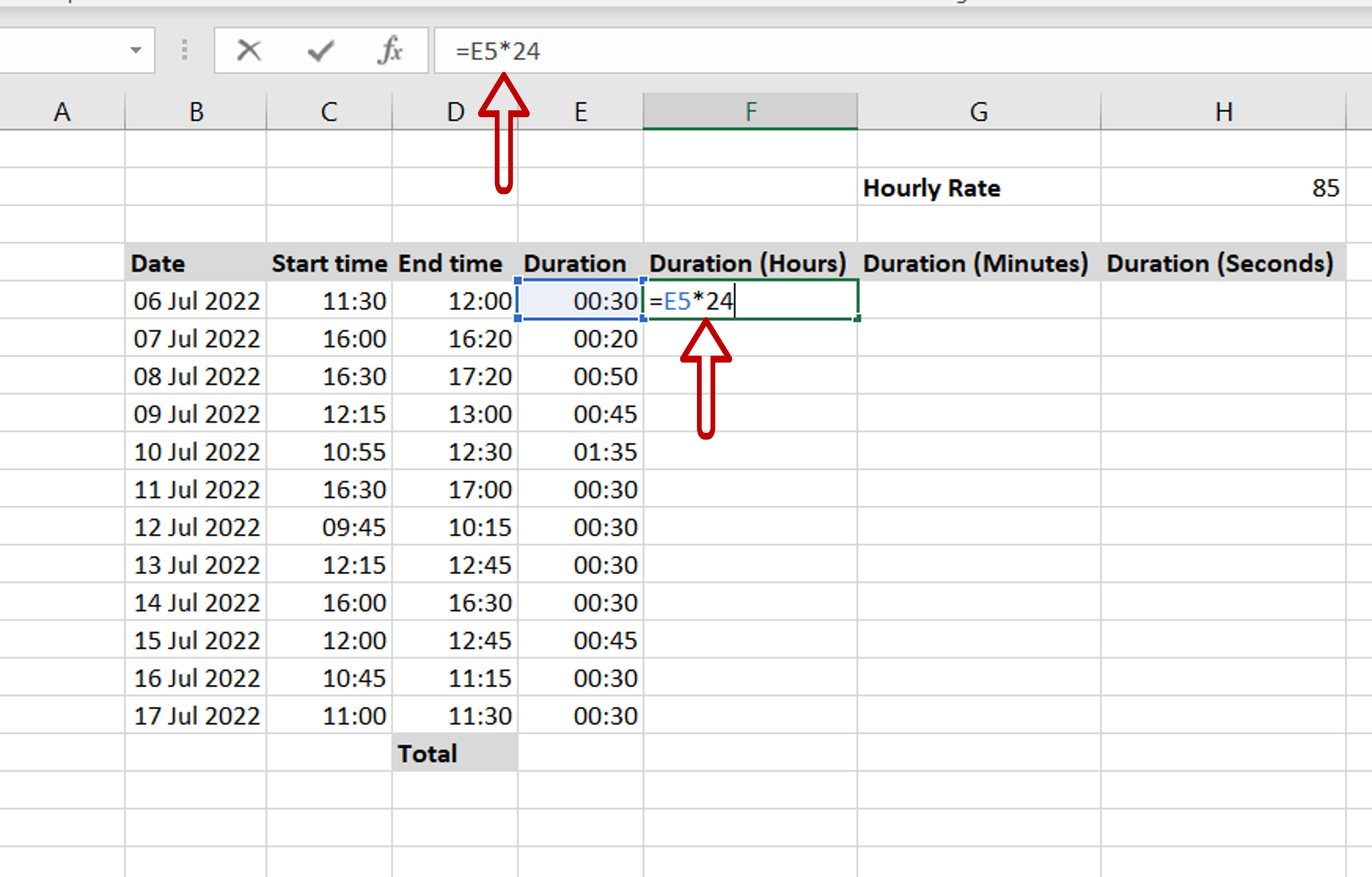– Select the destination cell
– Enable the cell for editing by pressing F2 or type the formula in the Formula bar
– Multiply the value to be converted by 24

### Step 2 – Open the Format Cells menu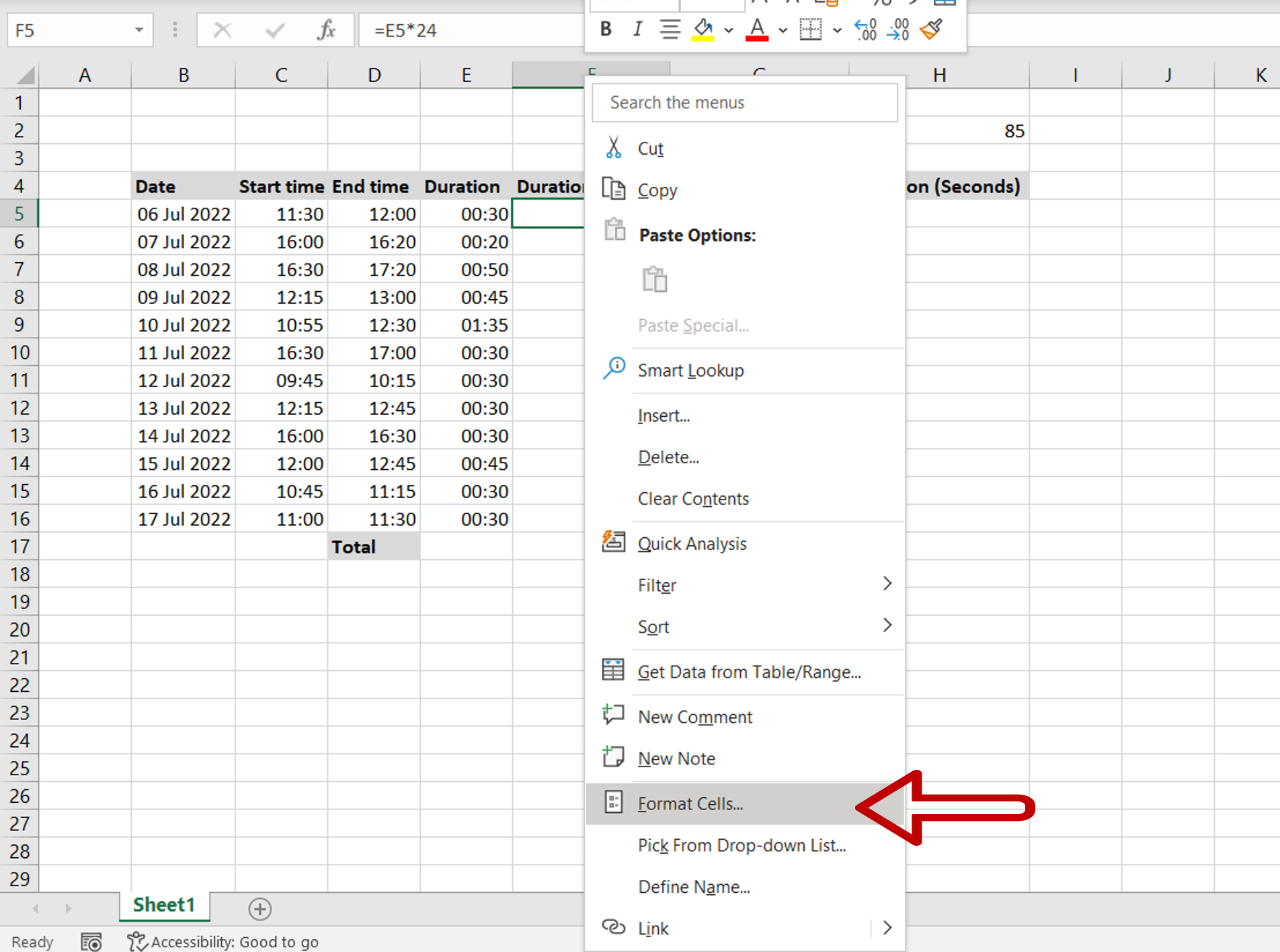– Right-click the cell and select Format Cells from the drop-down menu that appears
OR
– Go to Home > Cells > Format > Format Cells

### Step 3 – Format the cell as a number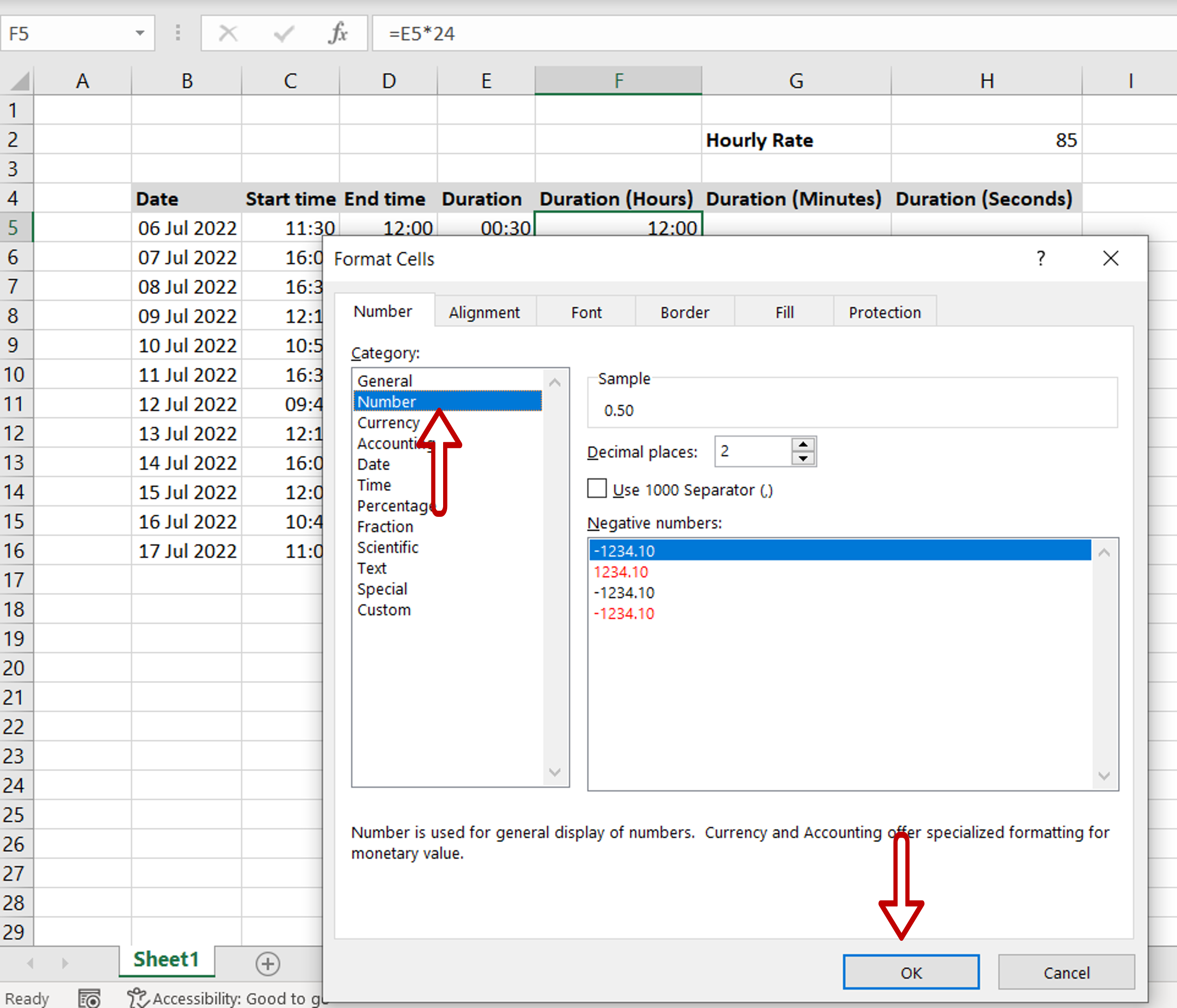– Select the number option

### Step 4 – Check the value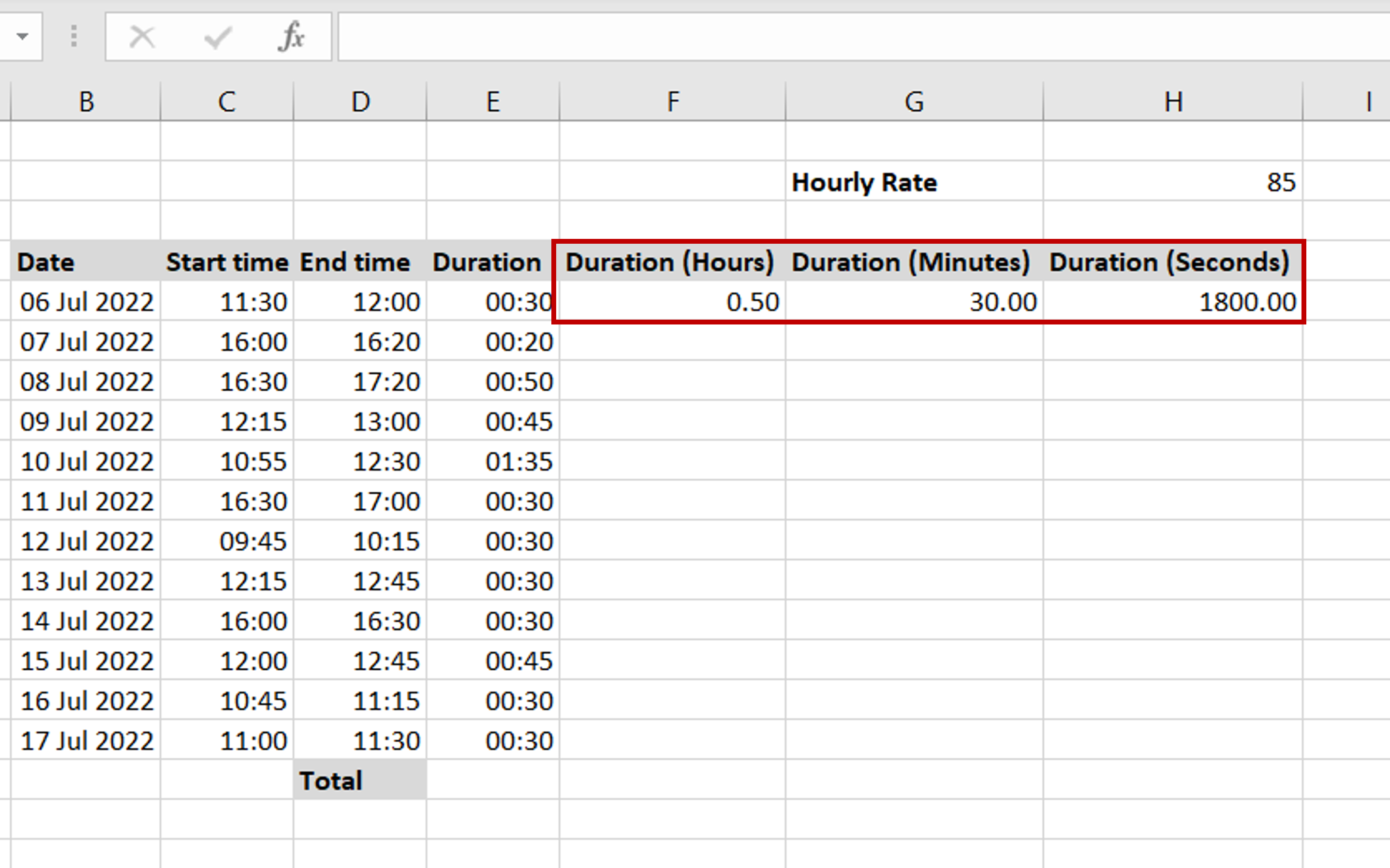– Multiplying by 24 converts the time into hours
– To convert to minutes, multiply by 24*60 i.e. 1,440
– To convert to seconds, multiply by 24*60*60 i.e. 86,400